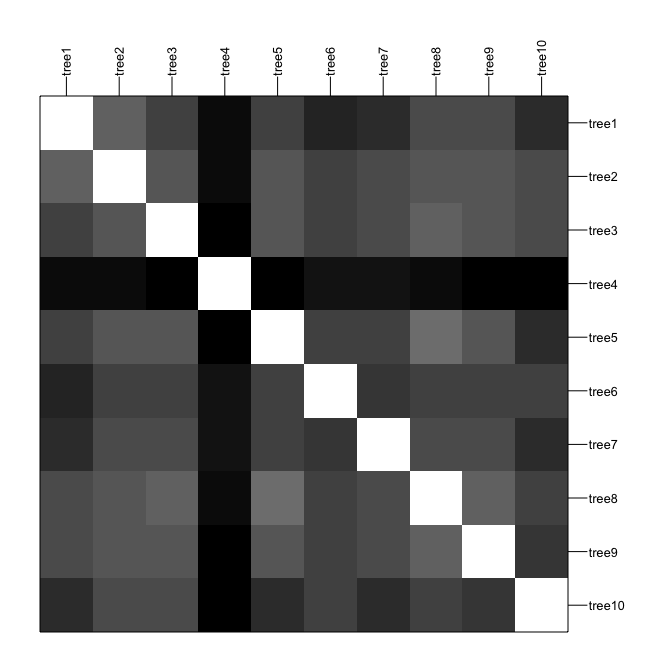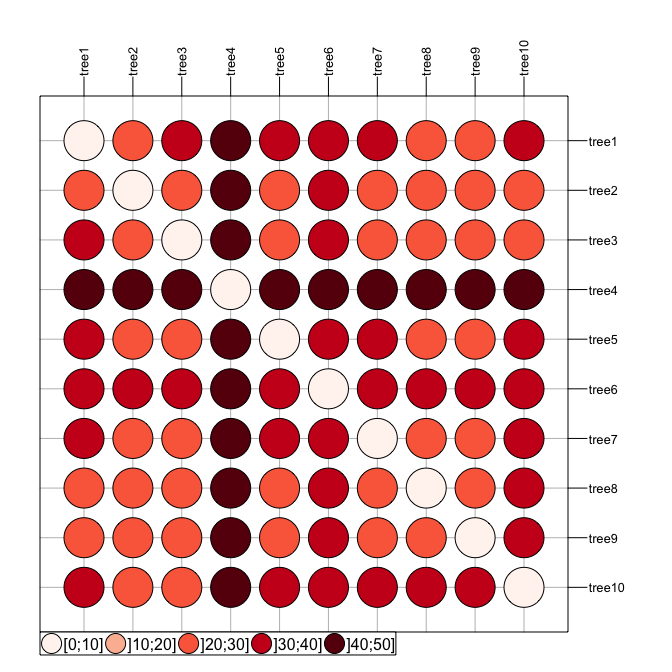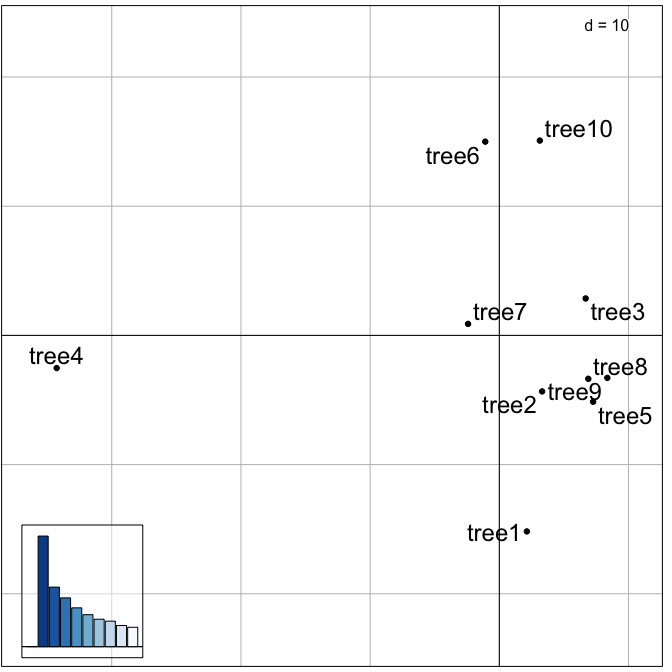# Exploration of landscapes of phylogenetic trees

#### 2018-05-14

treespace implements new methods for the exploration and analysis of distributions of phylogenetic trees for a given set of taxa.

## Installing treespace

To install the development version from github:

``````library(devtools)
install_github("thibautjombart/treespace")``````

The stable version can be installed from CRAN using:

``install.packages("treespace")``

Then, to load the package, use:

``library("treespace")``

## Content overview

The main functions implemented in treespace are:

• `treespace`: explore landscapes of phylogenetic trees

• `treespaceServer`: open up an application in a web browser for an interactive exploration of the diversity in a set of trees

• `findGroves`: identify clusters of similar trees

• `plotGroves`: scatterplot of groups of trees, and `plotGrovesD3` which enables interactive plotting based on d3.js

• `medTree`: find geometric median tree(s) to summarise a group of trees

Other functions are central to the computations of distances between trees:

• `treeVec`: characterise a tree by a vector

• `treeDist`: find the distance between two tree vectors

• `multiDist`: find the pairwise distances of a list of trees

• `refTreeDist`: find the distances of a list of trees from a reference tree

• `tipDiff`: for a pair of trees, list the tips with differing ancestry

• `plotTreeDiff`: plot a pair of trees, highlighting the tips with differing ancestry

Distributed datasets include:

• `woodmiceTrees`: illustrative set of 201 trees built using the neighbour-joining and bootstrapping example from the woodmice dataset in the ape documentation.

• `DengueTrees`: 500 trees sampled from a BEAST posterior set of trees from (Drummond and Rambaut, 2007)

• `DengueSeqs`: 17 dengue virus serotype 4 sequences from (Lanciotti et al., 1997), from which the `DengueTrees` were inferred.

• `DengueBEASTMCC`: the maximum clade credibility (MCC) tree from the `DengueTrees`.

## Exploring trees with treespace

We first load treespace, and the packages required for graphics:

``````library("treespace")
library("rgl")``````

The function `treespace` defines typologies of phylogenetic trees using a two-step approach:

1. perform pairwise comparisons of trees using various (Euclidean) metrics; by default, the comparison uses the Kendall and Colijn metric (Kendall and Colijn, 2016) which is described in more detail below; other metrics rely on tip distances implemented in adephylo (Jombart et al., 2010) and phangorn (Schliep 2011).

2. use Metric Multidimensional Scaling (MDS, aka Principal Coordinates Analysis, PCoA) to summarise pairwise distances between the trees as well as possible into a few dimensions; the output of the MDS is typically visualised using scatterplots of the first few Principal Components (PCs); this step relies on the PCoA implemented in ade4 (Dray and Dufour, 2007).

The function `treespace` performs both tasks, returning both the matrix of pairwise tree comparisons (`\$D`), and the PCoA (`\$pco`). This can be illustrated using randomly generated trees:

``````# generate list of trees
set.seed(1)
x <- rmtree(10, 20)
names(x) <- paste("tree", 1:10, sep = "")

# use treespace
res <- treespace(x, nf=3)
names(res)``````
``##  "D"   "pco"``
``res``
``````## \$D
##        tree1 tree2 tree3 tree4 tree5 tree6 tree7 tree8 tree9
## tree2  26.00
## tree3  31.06 26.74
## tree4  42.85 42.12 44.44
## tree5  30.66 27.71 27.37 44.79
## tree6  36.50 31.18 30.18 41.81 31.59
## tree7  34.64 28.71 29.48 40.35 31.11 32.37
## tree8  28.97 26.29 24.45 43.74 23.47 30.41 29.00
## tree9  29.63 27.42 27.48 45.61 26.31 30.89 29.77 24.60
## tree10 34.87 30.00 29.44 44.97 34.06 31.05 34.41 31.54 32.59
##
## \$pco
## Duality diagramm
## class: pco dudi
## \$call: dudi.pco(d = D, scannf = is.null(nf), nf = nf)
##
## \$nf: 3 axis-components saved
## \$rank: 9
## eigen values: 142.1 76.52 62.69 49.88 41.07 ...
##   vector length mode    content
## 1 \$cw    9      numeric column weights
## 2 \$lw    10     numeric row weights
## 3 \$eig   9      numeric eigen values
##
##   data.frame nrow ncol content
## 1 \$tab       10   9    modified array
## 2 \$li        10   3    row coordinates
## 3 \$l1        10   3    row normed scores
## 4 \$co        9    3    column coordinates
## 5 \$c1        9    3    column normed scores
## other elements: NULL``````

Pairwise tree distances can be visualised using adegraphics:

``````# table.image
table.image(res\$D, nclass=30)````````````# table.value with some customization
table.value(res\$D, nclass=5, method="color",
symbol="circle", col=redpal(5))``````The best representation of these distances in a 2-dimensional space is given by the first 2 PCs of the MDS. These can be visualised using any scatter plotting tool; here we use the treespace function `plotGroves`, based on the adegraphics function `scatter`:

``plotGroves(res\$pco, lab.show=TRUE, lab.cex=1.5)``Alternatively, `plotGrovesD3` creates interactive plots based on d3.js:

``plotGrovesD3(res\$pco, treeNames=1:10)``

Tree labels can be dragged into new positions to avoid problems such as overlapping.

The functionality of `treespace` can be further illustrated using ape’s dataset woodmouse, from which we built the 201 trees supplied in `woodmiceTrees` using the neighbour-joining and bootstrapping example from the ape documentation.

``````data(woodmiceTrees)
wm.res <- treespace(woodmiceTrees,nf=3)

# PCs are stored in:
``````##         A1     A2      A3
## 1  -0.9949 -1.363 -0.7918
## 2  -0.6137 -1.014 -0.6798
## 3   2.6667  4.219 -2.9293
## 4 -13.6081  1.854  1.0947
## 5   2.1980  4.176 -3.1960
## 6   3.6013  4.865  2.9853``````
``````# plot results
plotGrovesD3(wm.res\$pco)``````

Packages such as adegraphics and ggplot2 can be used to make alternative plots, for example visualising the density of points within the space.

The treespace function `multiDist` simply performs the pairwise comparison of trees and outputs a distance matrix. This function may be preferable for large datasets, and when principal co-ordinate analysis is not required. It includes an option to save memory at the expense of computation time.

## Identifying clusters of trees

Once a typology of trees has been derived using the approach described above, one may want to formally identify clusters of similar trees. One simple approach is:

1. select a few first PCs of the MDS (retaining signal but getting rid of random noise)

2. derive pairwise Euclidean distances between trees based on these PCs

3. use hierarchical clustering to obtain a dendrogram of these trees

4. cut the dendrogram to obtain clusters

In treespace, the function `findGroves` implements this approach, offering various clustering options (see `?findGroves`). Here we supply the function with our `treespace` output `wm.res` since we have already calculated it, but it is also possible to skip the steps above and directly supply `findGroves` with a multiPhylo list of trees.

``````wm.groves <- findGroves(wm.res, nclust=6)
names(wm.groves)``````
``##  "groups"    "treespace"``

Note that when the number of clusters (`nclust`) is not provided, the function will display a dendrogram and ask for a cut-off height.

The results can be plotted directly using `plotGrovesD3` (see `?plotGrovesD3` for options):

``````# basic plot
plotGrovesD3(wm.groves)``````
``````# alternative with improved legend and tooltip text, giving the tree numbers:
plotGrovesD3(wm.groves, tooltip_text=paste0("Tree ",1:201), legend_width=50, col_lab="Cluster")``````
``````# plot axes 2 and 3. This helps to show why, for example, clusters 2 and 4 have been identified as separate, despite them appearing to overlap when viewing axes 1 and 2.
plotGrovesD3(wm.groves, xax=2, yax=3, tooltip_text=paste0("Tree ",1:201), legend_width=50, col_lab="Cluster")``````

We can also plot in 3D:

``````# prepare a colour palette:
colours <- fac2col(wm.groves\$groups, col.pal=funky)
plot3d(wm.groves\$treespace\$pco\$li[,1],
wm.groves\$treespace\$pco\$li[,2],
wm.groves\$treespace\$pco\$li[,3],
col=colours, type="s", size=1.5,
xlab="", ylab="", zlab="")``````